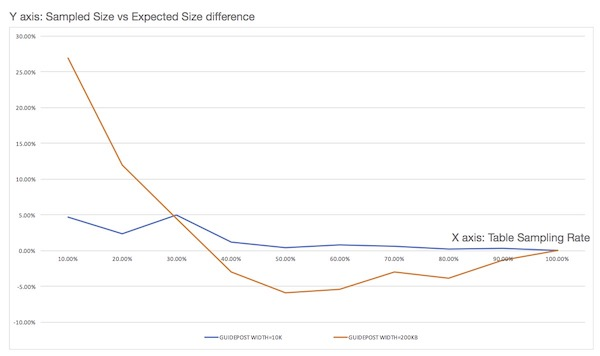To support table sampling, similar to the Postgresql and T-SQL syntax, a TABLESAMPLE clause has been encorporated into the table aliases ref as of Phoenix 4.12. The general syntax is described here. This feature limits the number of rows returned from a table to a PERCENT of rows. See PHOENIX-153 for implementation details.

This feature is implmented with a Bernoulli trial, a consistent-hashing based tablesampler, to achieve a Bernoulli sampling on a given population (rows). When executed, given a sampling rate, it leverages Phoenix’s stats as well HBase’s region distribution to perform a table sampling.

As part of the statistics collection, a guide post (a row referrence) is created for rows at equidistant bytes from each other. When sampling is required, a Bernoulli trial process is applied repeatedly on each guide post on each region with a probability that porportional to the sampling rate. A included guide post will result in all the rows in between this and next guide post being included into sample population.

#### Performance

Sampling on a table with a sampling rate = 100% will cost same amount of computational resource as a query without sampling. This resouces consumption quicky fall off when a smaller sampling rate is choosing. In general, the amortized complexity for sampling process is O(k + mn) , with n being the number of the regions of the sampled hbase table, m being the number of guide posts, and k being the size of sampled population.

#### Repeatable

Repeatable means it guarantees that the repeated sampling on the same table will resulted in the same sampled result.

Repeatable feature is made possible by a consistent-hashing process applied on the binary representation of the start rowkey of each guideposts of each region when sampling process is in action. During hashing, a FNV1 implementation with lazy mod method is adopted by default. see FNV1.

## Examples

For example, to tablesample a table , you would execute the following command. Please note, the sampling rate is a numeric value between 0 and 100, inclusive.

```select * from PERSON TABLESAMPLE(12.08);
```

Some more examples:

```select * from PERSON TABLESAMPLE (12.08) where ADDRESS = 'CA' OR name>'aaa';
select count( * ) from PERSON TABLESAMPLE (12.08) LIMIT 2
select count( * ) from (select NAME from PERSON TABLESAMPLE (49) limit 20)
select * from (select /+NO_INDEX/ * from PERSON tablesample (10) where Name > 'tina10') where ADDRESS = 'CA'
select * from PERSON1, PERSON2 tablesample (70) where PERSON1.Name = PERSON2.NAME
select /+NO_INDEX/ count( * ) from PERSON tableSample (19), US_POPULATION tableSample (28) where PERSON.Name > US_POPULATION.STATE
```

To use it in aggregation:

```select count( * ) from PERSON TABLESAMPLE (49) LIMIT 2
select count( * ) from (select NAME from PERSON TABLESAMPLE (49) limit 20)
```

To explain the query:

```explain select count( * ) from PERSON TABLESAMPLE (49) LIMIT 2)
```

## Tuning

• Due to sampling process, the TABLESAMPLE clause should be used with some caution. For example, a join of two tables is likely to return a match for each row in both tables; however, when tablesampling process is applied on either one or both of the two tables, the joining may not match the expectation when without sampling.

• The stats needs to be collected in order to achieve the best sampling accuracy. To turn on statistics collection, refer to Statistics Collection.

`  ALTER TABLE my_table SET GUIDE_POSTS_WIDTH = 10000000  `
* A denser guide post setting improves the sampling accuracy, but reduces the performance at the same time. A comparison is experimented as fig below: